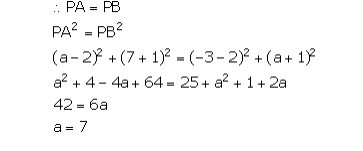Selina solutionsOur top 5% students will be awarded a special scholarship to Lido.
Set your child up for success with Lido, book a class today!

A point P (2, -1) is equidistant from the points (a, 7) and (-3, a). Find a.

• Solution

• Transcript

Point P (2, -1) is equidistant from the points A (a, 7) and B (-3, a)."hello students i am rita your math leader tutor and the today's question is a point p 2 comma minus 1 is equal distance from the points e comma 7 and minus 3 comma a find a so in this question we have to find the value of a and it is given that the p point that is 2 comma minus 1 is equidistance from point a and from point b now the coordinates of point a is a comma seven and the coordinates of point b is minus three comma a and their distance r equal it means ba distance is equal to p b the distance from point p to a and from point p to v is equal so now we apply the distance formula here now if p a is equal to p b then p a square is also equal to p b square now we apply the distance formula between pa then we got 2 minus a square plus 2 minus a just wait a minute if we can write this as pa it means a minus 2 square plus 7 minus 1 so minus minus become plus 1 square is equal to pp it means minus 3 minus 2 square plus 8 minus 1 so minus minus become plus 1 square now we solve this equation and get the value of a first of all we apply the identity of a minus b whole square that is a square plus b square minus 2 ap 7 plus 1 8 and 8 square is 64. minus minus plus 3 plus 2 5 and 5 square is 25 now we apply the identity a plus 1 b a plus 1 whole square that is a plus b whole square a square plus b square plus 2 ap when we solve this equation then we got a square minus a square plus 4 plus 64 then we got plus 60 8 and that is equal to and this is minus and we when minus is when plus a square come back to the left hand side then it becomes minus a square is equal to 25 plus 1 that is 26 plus 2 a and minus 4 a when goes back when goes to the right hand side then it becomes plus 4 a so a square a square cancel out 68 minus 26 is equal to 4 plus 2 6 a so 28 minus 26 is 42 is equal to 6 a and 42 divided by 6 is equal to a it in 6 on the 6 6 7 42 now the value of a is equal to 7 so this is our answer i hope you like this video so please subscribe leto for more updates and do comment your question thank you"

Our top 5% students will be awarded a special scholarship to Lido.Lido

Courses

Teachers

Book a Demo with us

Syllabus

Science
English
Coding

Terms & Policies

NCERT Question Bank

Maths
Science

Selina Question Bank

Maths
Physics
Biology

Allied Question Bank

Chemistry